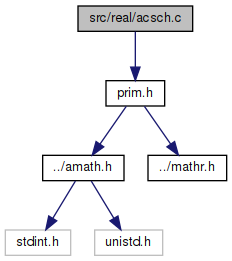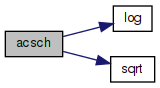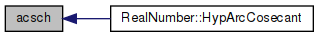amath  1.8.5 Simple command line calculator
acsch.c File Reference

Inverse hyperbolic cosecant function. More...

`#include "prim.h"`
Include dependency graph for acsch.c:Go to the source code of this file.

## Functions

double acsch (double x)
Inverse hyperbolic cosecant function. More...

## Detailed Description

Inverse hyperbolic cosecant function.

Definition in file acsch.c.

## ◆ acsch()

 double acsch ( double x )

Inverse hyperbolic cosecant function.

```Method
1+sqrt(1+x*x)
acsch(x) = ln( ------------— )
x
when x is 0
acsch(x) = NaN
```

Definition at line 49 of file acsch.c.

References log(), and sqrt().

Referenced by RealNumber::HypArcCosecant().

50 {
51  double a, b, c, d, e, f;
52
53  if (TRIG_INEXACT(x))
54  {
55  return NAN;
56  }
57
58  a = x * x;
59  b = a + 1.0;
60  c = sqrt(b);
61  d = 1.0 + c;
62  e = d / x;
63  f = log(e);
64  return f;
65 }
#define TRIG_INEXACT(x)
Definition: prim.h:48
#define NAN
Definition: mathr.h:53
double sqrt(double x)
Square root function.
Definition: sqrt.c:119
double log(double x)
Natural logarithm function (base e)
Definition: log.c:109
Here is the call graph for this function:Here is the caller graph for this function: